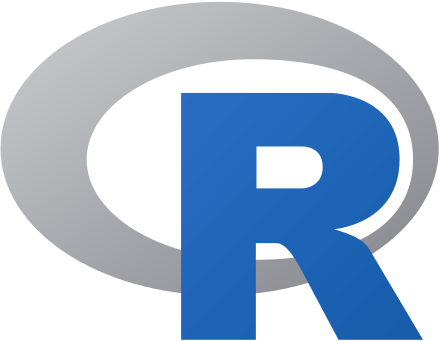You can find the entry of R as a programming language here in Wikipedia.

#### General information

Attribute In case of R
Developer R Core Team
First appeared August 1993; 25 years ago
Stable release 3.5.3 (“The Great Truth”)
Website www.r-project.org

#### Basic syntax

• The following examples illustrate the basic syntax of
• the language and
• use of the command-line interface.
• In R, the generally preferred assignment operator is an arrow made from two characters <-, although = can usually be used instead.
x <- 1:6  # Create vector.
y <- x^2  # Create vector by formula.
mean(y)  # Arithmetic mean of vector.
##  15.16667

The mean of vector y is 15.1666667.

The following code chunk is syntactically incorrect and thus should not be executed!

mean[y]

Last but not least - the well known R icon (from the wikipedia page):